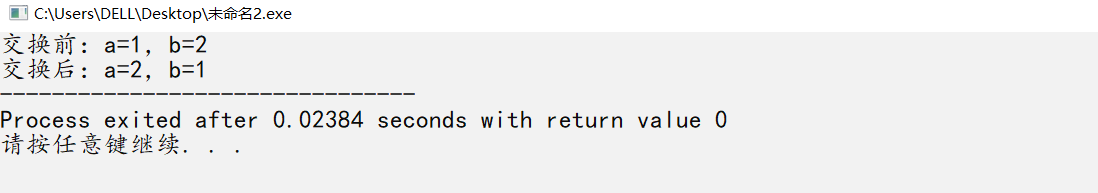• 交换两个变量的，不使用第三个变量的四种法方
千次阅读 多人点赞
2019-09-13 18:42:27

## 交换两个变量的值，不使用第三个变量的四种法方

通常我们的做法是（尤其是在学习阶段）：定义一个新的变量，借助它完成交换。代码如下：
int a,b;
a=10; b=15;
int t;
t=a; a=b; b=t;
这种算法易于理解，特别适合帮助初学者了解计算机程序的特点，是赋值语句的经典应用。在实际软件开发当中，此算法简单明了，不会产生歧义，便于程序员之间的交流，一般情况下碰到交换变量值的问题，都应采用此算法（以下称为标准算法）。

上面的算法最大的缺点就是需要借助一个临时变量。那么不借助临时变量可以实现交换吗？答案是肯定的！这里我们可以用三种算法来实现：1）算术运算；2）指针地址操作；3）位运算；4）栈实现。

1） 算术运算
int a,b;
a=10;b=12;
a=b-a; //a=2;b=12
b=b-a; //a=2;b=10
a=b+a; //a=10;b=10
它的原理是：把a、b看做数轴上的点，围绕两点间的距离来进行计算。
具体过程：第一句“a=b-a”求出ab两点的距离，并且将其保存在a中；第二句“b=b-a”求出a到原点的距离（b到原点的距离与ab两点距离之差），并且将其保存在b中；第三句“a=b+a”求出b到原点的距离（a到原点距离与ab两点距离之和），并且将其保存在a中。完成交换。
此算法与标准算法相比，多了三个计算的过程，但是没有借助临时变量。（以下称为算术算法）
缺点：是只能用于数字类型，字符串之类的就不可以了。a+b有可能溢出(超出int的范围)，溢出是相对的， +了溢出了，-回来不就好了，所以溢出不溢出没关系，就是不安全。

2） 指针地址操作
因为对地址的操作实际上进行的是整数运算，比如：两个地址相减得到一个整数，表示两个变量在内存中的储存位置隔了多少个字节；地址和一个整数相加即“a+10”表示以a为基地址的在a后10个a类数据单元的地址。所以理论上可以通过和算术算法类似的运算来完成地址的交换，从而达到交换变量的目的。即：
int a,b; //假设
a=new int(10);
b=new int(20); //&a=0x00001000h,&b=0x00001200h
a=(int
)(b-a); //&a=0x00000200h,&b=0x00001200h
b=(int
)(b-a); //&a=0x00000200h,&b=0x00001000h
a=(int
)(b+int(a)); //&a=0x00001200h,&b=0x00001000h
通过以上运算a、b的地址真的已经完成了交换，且a指向了原先b指向的值，b指向原先a指向的值了吗？上面的代码可以通过编译，但是执行结果却令人匪夷所思！原因何在？
首先必须了解，操作系统把内存分为几个区域：系统代码/数据区、应用程序代码/数据区、堆栈区、全局数据区等等。在编译源程序时，常量、全局变量等都放入全局数据区，局部变量、动态变量则放入堆栈区。这样当算法执行到“a=(int
)(b-a)”时，a的值并不是0x00000200h，而是要加上变量a所在内存区的基地址，实际的结果是：0x008f0200h，其中0x008f即为基地址，0200即为a在该内存区的位移。它是由编译器自动添加的。因此导致以后的地址计算均不正确，使得a,b指向所在区的其他内存单元。再次，地址运算不能出现负数，即当a的地址大于b的地址时，b-a<0，系统自动采用补码的形式表示负的位移，由此会产生错误，导致与前面同样的结果。
有办法解决吗？当然！以下是改进的算法：
if(a<b)
{
a=(int*)(b-a);
b=(int*)(b-(int(a)&0x0000ffff));
a=(int*)(b+(int(a)&0x0000ffff));
}
else
{
b=(int*)(a-b);
a=(int*)(a-(int(b)&0x0000ffff));
b=(int*)(a+(int(b)&0x0000ffff));
}
算法做的最大改进就是采用位运算中的与运算“int(a)&0x0000ffff”，因为地址中高16位为段地址，后16位为位移地址，将它和0x0000ffff进行与运算后，段地址被屏蔽，只保留位移地址。这样就原始算法吻合，从而得到正确的结果。
此算法同样没有使用第三变量就完成了值的交换，与算术算法比较它显得不好理解，但是它有它的优点即在交换很大的数据类型时，它的执行速度比算术算法快。因为它交换的时地址，而变量值在内存中是没有移动过的。（以下称为地址算法）

3） 位运算
int a=10,b=12; //a=1010^b=1100;
a=a^b; //a=0110^b=1100;
b=a^b; //a=0110^b=1010;
a=a^b; //a=1100=12;b=1010;
此算法能够实现是由异或运算的特点决定的，通过异或运算能够使数据中的某些位翻转，其他位不变。这就意味着任意一个数与任意一个给定的值连续异或两次，值不变。

4）栈实现。不多解释了，栈和相关函数定义省去。
int exchange(int x,int y)
{
stack S;
push(S,x);
push(S,y);
x=pop(S);
y=pop(S);
}

以上算法均实现了不借助其他变量来完成两个变量值的交换，相比较而言算术算法和位算法计算量相当，地址算法中计算较复杂，却可以很轻松的实现大类型（比如自定义的类或结构）的交换，而前两种只能进行整形数据的交换（理论上重载“^”运算符，也可以实现任意结构的交换）。
介绍这三种算法并不是要应用到实践当中，而是为了探讨技术，展示程序设计的魅力。从中可以看出，数学中的小技巧对程序设计而言具有相当的影响力，运用得当会有意想不到的神奇效果。而从实际的软件开发看，标准算法无疑是最好的，能够解决任意类型的交换问题。

更多相关内容
• 以上这篇Python3之不使用第方变量,实现交换个变量就是小编分享给大家的全部内容了，希望能给大家一个参考，也希望大家多多支持软件开发网。 您可能感兴趣的文章:python交换个变量方法对Python中实现...
• 本篇文章是对在php中交换个变量的方法进行了详细的分析介绍，需要的朋友参考下
• 相信每个刚接触PHP语言的童鞋们应该藕知道，PHP中两个变量的值互换是个很常见的做法，那么下面这篇文章我们就来介绍几个对于变量值互换的常见操作方法，文中介绍的很详细，相信对于大家的理解和学习很有帮助，下面有...
• 这篇文章主要介绍了JavaScript交换个变量方法实例,文中通过示例代码介绍的非常详细，对大家的学习或者工作具有一定的参考学习价值,需要的朋友可以参考下 在平时的业务开发或者面试过程中，经常会遇到交换个变量...
• 在一些公司的面试中可能会有些面试官会出一些奇奇怪怪的问题，比如怎么样计算1加到100的和要求不能使用加法，减法，循环和if判断，再比如怎么样不用第三个变量交换两个变量的等问题。这里我们先分析下怎么样不用...

## 前言

在一些公司的面试中可能会有些面试官会出一些奇奇怪怪的问题，比如怎么样计算1加到100的和要求不能使用加法，减法，循环和if判断，再比如怎么样不用第三个变量来交换两个变量的值等问题。这里我们先分析下怎么样不用第三个变量来交换两个变量的值。

## 正文

通常情况下我们会用第三个变量来进行两个变量的交换，比如在冒泡排序中会经常用到。那么怎么样不用第三个变量而只通过两个变量本身来进行交换呢？

swap(a,b); //C++自带的一个交换函数

swap()这个函数在C++中可以直接用来交换两个变量，使用很方便，但是如果不能用自带函数呢？

a=a+b;//a的值变成了a+b的和
b=a-b;//相当于a+b-b，结果就是原来a的值赋值给了b
a=a-b;//相当于a+b-a，结果就是把原来的b的值赋值给了a

这种办法很巧妙的对两个变量进行了交换，而不用第三个变量，但是需要注意的是变量的范围，比如这两个变量都是int，而int的取值范围是~，如果a这个时候刚好是，那么再加上b是不是就超过了int的范围了？是不是就无法完成了交换了？不会。因为当cpu计算完a+b的时候这个结果会缓存在cache中，当下次要继续用这个结果的时候会直接从cache中读取，而不会再次从内存中读取，这样的读取方式能很大程度上提高程序的运行速度。

展开全文C++
• 代码如下: //by ppchen var a = 10, b = 2; a = a + b; b = a – b; a = a – b; 代码如下: //by Mr.... b = b^a; a = a ^b; 代码如下: //by cloudgamer var... 评：1、2种解法是普遍解，只能交换数字，而3、4种则适用性更
• 主要介绍了在JS中 实现不用中间变量temp 实现两个变量值得交换 ,需要的朋友可以参考下
• #include<stdio.h>... printf("交换前：a=%d，b=%d\n",a,b); a=a+b;//a变为两项之和，b没变 b=a-b;//b得到原来的a a=a-b;//a得到原来的b printf("交换后：a=%d，b=%d",a,b); return 0; }

1.通过两变量之间相加减交换值。（如果int值过大，可能存在溢出问题）

#include<stdio.h>
int main()
{
int a=1;
int b=2;
printf("交换前：a=%d，b=%d\n",a,b);
a=a+b;//a变为两项之和，b没变
b=a-b;//b得到原来的a
a=a-b;//a得到原来的b
printf("交换后：a=%d，b=%d",a,b);
return 0;
}

2.通过位异或（^）办法交换值。（不会溢出）

^ ——按（二进制）位异或，相同为0，不同为1。（异或异为真）

二进制中a=01，b=10，每位进行异或比较，得到结果。

#include<stdio.h>
int main()
{
int a=1;//a=01
int b=2;//b=10
printf("交换前：a=%d，b=%d\n",a,b);
a=a^b;//a=11
b=a^b;//b=01
a=a^b;//a=10
printf("交换后：a=%d，b=%d",a,b);
return 0;
}一般情况下会使用第三个变量的方法交换两变量的值，代码可读性高，执行效率高；异或操作可读性差，执行效率低于其他方法！

展开全文c语言 开发语言
• 也是说，通过某种运算（二元运算）将a和b两个数变成一个数，并保存在其中一个变量中。然后再通过同 样的运算符将a或b中和掉。这样实际上是利用了a或 b本身作为了中间变量。 　先看第一个算法。 static　class　...
• ## 交换两个变量的值的三种方法

千次阅读 多人点赞 2021-03-06 19:04:20
方法一：定义临时变量法 运用此方法比较简单 public class 定义临时变量 { public static void main(String[] args) { int v1=1; int v2=2; System.out.println("v1="+v1+","+"v2=...//实现交换 v1=v2; v2=temp

## 方法一：定义临时变量法

运用此方法比较简单

public class 定义临时变量 {

public static void main(String[] args) {
int v1=1;
int v2=2;
System.out.println("v1="+v1+","+"v2="+v2);
int temp;//定义临时变量
temp=v1;//实现交换
v1=v2;
v2=temp;
System.out.println("v1="+v1+","+"v2="+v2);

}
}


代码运行结果如下:## 方法二：运算符法

运用此方法有好处也有弊端。
好处：不用再定义临时变量。
弊端：加法操作可能会导致超出存储范围，并且只能用于数值型变量的交换，有一定的局限性。

public class 运算符法 {
public static void main(String[] args) {
int v1=1;
int v2=2;
System.out.println("v1="+v1+","+"v2="+v2);
v1=v1+v2;//3
v2=v1-v2;//1
v1=v1-v2;//2
System.out.println("v1="+v1+","+"v2="+v2);
}

}


代码运行结果如下:## 方法三：位运算法（异或）

位运算符操作的都是整型的数据，所以此方法也具有一定的局限性。
异或运算法则：如果两个值不相同，则异或结果为1，如果两个值相同，异或结果为0。

public class 位运算法 {
public static void main(String[] args) {
int v1=1;
int v2=2;
System.out.println("v1="+v1+","+"v2="+v2);
v1=v1^v2;
v2=v1^v2;
v1=v1^v2;
System.out.println("v1="+v1+","+"v2="+v2);
}
}



代码运行结果如下:新手上路，因能力有限，若有不足之处还望大家海涵！

展开全文java
• 交换个变量 int a = 10; int b = 20; 方案A:使用第方变量（开发中使用） int c = a; a = b; b = c; 方案B:用异或的特点实现（面试使用） a = a ^ b; b = a ^ b;//a ^ b ^ b = a a = a ^ b;//a ^ b ^ a = b ...
• 大部分语言，例如c语言，交换个变量需要使用中间变量。例如交换a,b伪代码：tmp = aa = bb = tmppython里面可以实现无临时变量的交换(a,b) = (b,a)对于它的交换原理我深感好奇，因为这意味着python解释器很有...
• 这样可以先改变另一个变量值， 最后改变原修改的变量值 比如这个 a = a + b; b = a - b; a = a - b; 让a先变成a与b的‘和’（也可以换成a和b的差，一样的） ‘和’减去b巧妙的得到了a的变量值赋予b 再通过‘和’减去...
• java中交换2个变量值种方法 例：int a = 9;b = 1;交换a,b的值 第一种：生成第方变量（多占内存） int temp = a; //生成第方临时变量temp，将a的值赋给它 a = b; //将b的值赋给a b = temp; //将临时变量...java 算法
• 学过编程的人都知道，在交换个变量时，大多数人习惯定义一个临时变量来存放其中的一个，防止交换数值时其中一个变量被覆盖掉，比如下面的一段用C++写的很简单的程序：     /**交换个变量...
• 方法1：实现两个变量值交换，不使用第三个变量 我们可以通过数学运算中的差值来实现交换   int a=10,b=20;     a=a+b;     b=a-b;     a=a-b;     交换成功，运行结果如下图 ...
• 刚学编程的时候大家肯定都知道，可以用第三个变量做中间变量实现两个变量的交换 c=a; a=b; b=c; 那么如果不使用这个变量c呢？ 今天学习了两种方法，第一种如下： a=a+b； b=a-b； a=a-b； 这种方法比较好理解。...
• 这种方法很容易理解，就是创建temp中间变量，m把给temp，n给m，temp将m的给n，这样就实现了交换。 2.加减运算（前提只能是数字） 因为这种方法需要运算，所有只能是数字，eg：m=8，n=2；第一步求出两数的...
• b=12,要求在不使用第三个变量的情况下相互交换值。 下面是个人愚见，欢迎吐槽~~ $a = 10;$b = 12; //运用算法 $a =$a + $b;$b = $a-$b; $a =$a-$b; //字符串截取法$len_a = strlen($a);$a = $a .$b; \$b ...
• 如何在不引入其他变量的情况下，交换变量a和b的嘞？ 例如：a=2，b=4，交换使得：a=4,b=2，如何解决？？？？？ 方法一：利用异或运算（/\） 异或运算符：/\，运算规则：若参加运算的两二进制相同则为0，不同则...位运算
• 不借用第三个变量，如何交换两个变量的？ 算术运算 int exchange(int x,int y)  {  stack S;  push(S,x);  push(S,y);  x=pop(S);  y=pop(S);  }
• 种方法交换个变量 文章目录种方法交换个变量1. 加减法2. 异或法3. 交换变量法 1. 加减法 #include &lt;stdio.h&gt; int main() { int a = 3; int b = 5; a = a + b; b = a - b; a ...
• 对于交换个变量,我们通常的操作都是,定义一个变量,借助它实现两个变量交换: int a = 1,b = 2; int c = a; a = b; b = c;这算是一种比较基础的实现,但是如果不许定义多余的变量呢?我们还有几种方法,下面...
• 今天看了一大佬的代码，发现了一这样的几行代码： arr[i]=arr[i]^arr; arr=arr[i]^arr; arr[i]=arr[i]^arr; 第一次见到这样的代码，不知道大佬在干什么。然后简单带入几二进制...
• python中交换个变量值的几种方式 1.python中独有的，也是最简单的方式： a,b=b,a 2.引入第三个变量，可以看作两个装满牛奶的瓶子a和瓶子b，如果我想交换这两个瓶子中的牛奶，就需要一个空瓶temp，先将a瓶中的...
• 交换个变量值，没有要求的时候原理为：定义一个中间变量，将a的值赋于中间变量，b的值赋于a，最后将中间变量的值赋于b即可 但是如果要求不能使用第变量来达到交换个变量值的目的呢？ 程序运行结果如下图：...